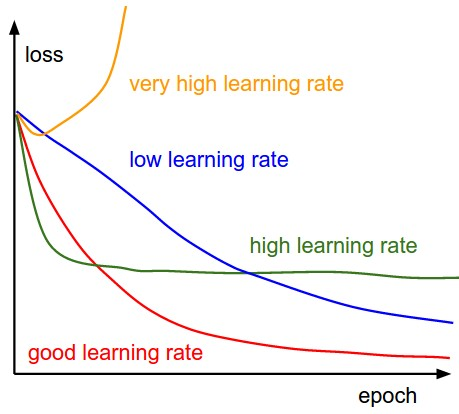###Author Topic: Train Loss & Learning rate (on YOLOv2 )  (Read 38 times)

0 Members and 1 Guest are viewing this topic.

####Flavio58##### Train Loss & Learning rate (on YOLOv2 )
« Reply #1 on: February 05, 2019, 04:05:05 AM »
Advertisement
Train Loss & Learning rate (on YOLOv2 )

During training any deep learning model, it is vital to look at the loss in order to get some intuition about how network (detector, classifier and etc.) is learning. For example, if you look at the Figure below, training loss for people detector that I am training already stopped decreasing even if it is … Continue reading Train Loss & Learning rate (on YOLOv2 )Credit to Standford cs231n course

During training any deep learning model, it is vital to look at the loss in order to get some intuition about how network (detector, classifier and etc.) is learning. For example, if you look at the Figure below, training loss for people detector that I am training already stopped decreasing even if it is only in the initial stages of the training. Usually, in the initial stages, it is common to see a loss decreasing very fast and smoothly.  Since that is not a case here, we can conclude that something is wrong here with Learning-Rate. Learning rate is the parameter that decides how big step network should take when searching for an optimal solution.Figure1

If your learning rate is big for your task(dataset) then the case something like below happens. Here network cannot make the small change that is needed to optimize because provided learning rate is too big.

So the loss function that varies a lot and does not decrease is an indication that our learning rate is big. For the training setting above my learning rate was learning_rate=0.0001  .

Let’s see what would happen if we increase learning rate 3x  (learning_rate=0.0003). Okay, let’s see what our loss function looks like when we have 3x bigger learning_rate (Figure 2).Figure2: Loss Function for the same architecture as above but 3x bigger learning_rate (learning_rate=0.0003)

Oh boy, that doesn’t look good, does it?  After 160 iterations it starts increasing and then later until 200 iteration network tries to go back to track to – search for the parameters that would minimize loss, but because of too big learning_rate it fails and loss starts increasing again after 200 iterations (Figure 2).

At around 290 iterations there is no point to continue because loss is going towards +infinity (Figure 3)Figure 3: Loss Function for the same architecture as in Figure 1 but 3x bigger learning rate (learning_rate=0.0003)

Takeaway lesson is: when you have slightly large learning_rate for your dataset/task then you see your loss will stop decreasing in the beginning of the training (Figure 1). But if you give too big learning_rate you will have a problem where your loss starts increasing instead of decreasing (Figure2, Figure3).

As we said above the problem in in Figure 1 was big learning rate, so to show you the big learning_rate problem, we tried 3x larger learning rate and see the loss in Figure 2 and Figure 3. Here in Figure 4 you see the the loss function when we provide 3x smaller learning rate compared initial learning_rate (learning_rate=0.0001). Our new learning_rate becomes new learning_rate = 1/3*0.0001 and when we train our network with this learning_rate we see stable loss decrease in the Figure 4 compared to in Figure 1.In practice, I will try 3-5 learning rate (for example 0.001,0.001*3,0.001*3*3,0.001*3*3). Train for about 1000 iterations, compare their loss, and choose the best learning rate to use during the whole training.

That concludes our explanation about Loss and Learning rate. BTW, the reason I am decreasing or increasing learning_rate by the factors of 3 is because it is  a rule of thumb in machine learning when searching for the right learning_rate to increase or decrease the learning_rate by factor of 3.

Source: Train Loss & Learning rate (on YOLOv2 )

Consulente in Informatica dal 1984

Software automazione, progettazione elettronica, computer vision, intelligenza artificiale, IoT, sicurezza informatica, tecnologie di sicurezza militare, SIGINT.

Facebook:https://www.facebook.com/flaviobernardotti58
Twitter : https://www.twitter.com/Flavio58

Cell:  +39 366 3416556

f.bernardotti@deeplearningitalia.eu

#deeplearning #computervision #embeddedboard #iot #ai

### Related Topics

Subject / Started by Replies Last post0 Replies
154 ViewsMarch 17, 2018, 02:26:01 PM
by Ruggero Respigo0 Replies
87 ViewsJune 07, 2018, 11:45:12 AM
by Ruggero Respigo0 Replies
55 ViewsMarch 01, 2019, 10:07:08 AM
by Flavio580 Replies
3 ViewsJune 26, 2019, 04:10:38 PM
by Flavio580 Replies
5 ViewsJune 30, 2019, 12:08:15 AM
by Flavio58